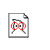# General Schema Theory for Genetic Programming with Subtree-Swapping Crossover

Created by W.Langdon from gp-bibliography.bib Revision:1.6717

@TechReport{Poli00-16,
• author = "Riccardo Poli",
• title = "General Schema Theory for Genetic Programming with Subtree-Swapping Crossover",
• institution = "University of Birmingham, School of Computer Science",
• number = "CSRP-00-16",
• month = nov,
• year = "2000",
• keywords = "genetic algorithms, genetic programming",
• email = "R.Poli@cs.bham.ac.uk",
• file = "/2000/CSRP-00-16.ps.gz",
• URL = "ftp://ftp.cs.bham.ac.uk/pub/tech-reports/2000/CSRP-00-16.ps.gz",
• abstract = "In this paper a new general schema theory for genetic programming is presented. Like other recent GP schema theory results, the theory gives an exact formulation (rather than a lower bound) for the expected number of instances of a schema at the next generation. The theory is based on a Cartesian node reference system which makes it possible to describe programs as functions over the space N^2 and allows one to model the process of selection of the crossover points of subtree-swapping crossovers as a probability distribution over N^4. The theory is also based on the notion of variable-arity hyperschema, which generalises previous definitions of schema or hyperschema introduced in GP. The theory includes two main theorems describing the propagation of GP schemata: a microscopic schema theorem and a macroscopic one. The microscopic version is applicable to crossover operators which replace a subtree in one parent with a subtree from the other parent to produce the offspring. Therefore, this theorem is equally applicable to standard GP crossover with and without uniform selection of the crossover points, as it is to one-point crossover, size-fair crossover, strongly-typed GP crossover, context-preserving crossover and many others. The macroscopic version is applicable to crossover operators in which the probability of selecting any two crossover points in the parents depends only on their size and shape. In the paper we provide examples which show how the theory can be specialised to specific crossover operators and how it can be used to derive other general results such as an exact definition of effective fitness and a size-evolution equation for GP with subtree-swapping crossover.",
}

Genetic Programming entries for Riccardo Poli